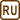## Inductance of a Coil

Inductance of a closely wound coil is such that when the current changes by 5 A per second, it induces electromotive force of 3 mV. Furthermore, we know that a steady current of 8 A generates in each loop of the coil a magnetic flux of 40 μWb.

a) Calculate the inductance of the coil.

b) Determine the number of loops of the coil.

• #### Hint

Find the equation describing electromotive force induced in a coil.

The inductance of a coil is a constant of proportionality between the current flowing through the coil and the magnetic induction flux through the coil.

• #### Analysis

a) Electric current flowing through one loop of the coil creates within this loop magnetic flux Φ, which is directly proportional to the current. Together, all of the N loops of the coil create overall flux . The electromotive force induced in the coil is equal to the rate of change of magnetic flux with respect to time. The overall flux can also be determined by the coil inductance and the current.

b) To calculate the number of loops of the coil, we use the relationship between the coil inductance and the total magnetic flux.

• #### Solution

a) Electromotive force Ui induced in the coil is proportional to the rate of change of the total magnetic flux

$|U_i| =\left| \frac {\Delta(N\Phi)}{\Delta t}\right|$

For the coil inductance L the following relation applies (see Hint)

$N \Phi = L I$

We combine both formulas and we obtain

$|U_i| = \frac {\Delta L I}{\Delta t}$

Inductance of the coil L is constant, and therefore in the formula there is the rate of change of current with respect to time, which we were given in the assignment

$|U_i| =L \frac {\Delta I}{\Delta t}$

From the last relation we evaluate the unknown inductance L.

$L=\dfrac{|U_i|}{\frac {\Delta I}{\Delta t}}$

b) From the relation for the coil inductance

$N \Phi = L I$

we evaluate the number of loops N

$N=\frac{LI}{\Phi}$
• #### Numerical values and insertion

$\frac{\Delta I}{\Delta t}= 5 \,\mathrm{As^{-1}}$ $|U_i| = 3\,\mathrm {mV}= 3 {\cdot} 10^{-3} \,\mathrm{V}$ $I=8\,\mathrm{A}$ $\Phi = 40\,\mathrm{\mu Wb}=40 {\cdot} 10^{-6}\,\mathrm{Wb}$ $L= \mathrm{?}$ $N=\mathrm{?}$
$L=\frac{|U_i|}{\frac{\Delta I}{\Delta t}}=\frac{3{\cdot} 10^{-3}}{5}\,\mathrm{H}= 6{\cdot} 10^{-4}\,\mathrm{H}=0.6\,\mathrm{mH}$ $N=\frac{LI}{\Phi}=\frac{6{\cdot} 10^{-4}\cdot 8}{40 {\cdot} 10^{-6}}= 120$